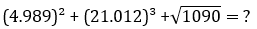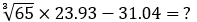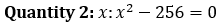### Quantitative Aptitude Quiz For NIACL AO

Numerical Ability or Quantitative Aptitude Section has given heebie-jeebies to the aspirants when they appear for a banking examination. As the level of every other section is only getting complex and convoluted, there is no doubt that this section, too, makes your blood run cold. The questions asked in this section are calculative and very time-consuming. But once dealt with proper strategy, speed, and accuracy, this section can get you the maximum marks in the examination. Following is the Quantitative Aptitude quiz to help you practice with the best of latest pattern questions.

Directions (1-5): Find the approximate value of the following questions

Q1. 12.002 × 15.005 – 8.895 × 6.965 = ?
130
117
105
110
95

Q2. 105.1% of 8401.01 – 3/7% of 5600.12 + 9.999 = ?
8880
8080
8850
8760
8806

Q3. 30.01² – 19.98² - ? = 21.97²
49
50
30
39
16

Q4.9219
9391
9319
9129
9643

Q5.98
65
102
35
79

Q6. A tradesman gives 4% discount on the marked price and gives 1 article free for buying every 15 articles and thus gains 35%. The marked price is above the cost price by :
40%
39%
20%
50%
None of these

Q7. Present age of Sunita is 3 times the present age of Nita. 4 years hence twice the age of Sunita will be equal to thrice the age of Nita. Find the present age of Sunita.
2 years
5 years
9 years
6 years
4 years

Q8. Average age of A, B and C is 10 year less than age of C. If ratio of age of A, B and C is 3 : 4 : 5 then what is the sum of age of A & C.
80 year
70 year
85 year
78 year
72 year

Q9. Simple interest on a certain sum at 7 p.c.p.a for 4 years is Rs. 3584. What will be the compound interest on the same principal at 4 p.c.p.a. in two years?
Rs. 1054.48
Rs. 1044.48
Rs. 938
Rs. 1064.84
None of these

Q10. There is some profit when an article is sold for Rs. 720. However when the same article is sold for Rs. 420, there is some loss. If the quantum of loss is two times the quantum of profit, find the cost price of the article.
Rs. 620
Rs. 700
Rs. 520
Rs. 840
Cannot be determined

Directions (11-15): In the following questions, two quantities (Quantity 1 and 2) are given. You have to find each quantity and compare both quantities as-

Q11. Quantity 1: Simple interest on the same sum for same 4 years at the same rate of interest. The simple interest on a certain sum at a certain rate of interest for two years is Rs. 3200 and compound interest on the same sum at the same rate of interest for two years is Rs. 3520.
Quantity 2: Total income of Mr. Bansal. Out of his total income, Mr. Bansal spends 25% on house rent and 80% of the rest on house hold expenses. he saves Rs 1,320.
Quantity 1 > Quantity 2
Quantity 1 < Quantity 2
Quantity 1 ≥ Quantity 2
Quantity 1 ≤ Quantity 2
Quantity 1 = Quantity 2 or relationship cannot be established.

Q12. Quantity 1: Value of k. A sum at R% compound interest doubles in 3 years. In 9 years it will be k times of the original principal.
Quantity 2: Rate percent per annum at which Rs. 3000 amounts to Rs. 3993 in 3 years, if the interest in compounded annually?
Quantity 1 > Quantity 2
Quantity 1 < Quantity 2
Quantity 1 ≥ Quantity 2
Quantity 1 ≤ Quantity 2
Quantity 1 = Quantity 2 or relationship cannot be established.

Q13. Quantity 1: Time taken by B to cover the same distance as that by C. A is twice as fast as B and B is thrice as fast as C. C covers the same journey in 54 minutes.
Quantity 2: Time for which bus is stopped per hour. Excluding stoppages, the speed of a bus is 54 kmph and including stoppages, it is 45 kmph.
Quantity 1 > Quantity 2
Quantity 1 < Quantity 2
Quantity 1 ≥ Quantity 2
Quantity 1 ≤ Quantity 2
Quantity 1 = Quantity 2 or relationship cannot be established.

Q14. Quantity 2: Initial quantity of mixture in the bucket. There is a certain quantity of a mixture of sugar cane and water in a bucket. Due to a leak in the bottom of bucket 8 litre of mixture were gone out. At the same time leak was observed, leak was closed and filled with same quantity of water. The original ratio of sugar cane to water and new ratio of sugar cane to water is 13: 7 and 13: 9 respectively.Quantity 1 > Quantity 2
Quantity 1 < Quantity 2
Quantity 1 ≥ Quantity 2
Quantity 1 ≤ Quantity 2
Quantity 1 = Quantity 2 or relationship cannot be established.

Q15. Quantity 1: No. of blue tiles. The length and breadth of the floor of a room are 20 feet and 10 feet respectively. Square tiles of 2 feet length of three different colors are to be laid to the floor. Black tiles are laid in the first row on all sides. If white titles are laid in the one-third of the remaining and blue tiles in the rest, how many blue tiles will be there?Quantity 1 > Quantity 2
Quantity 1 < Quantity 2
Quantity 1 ≥ Quantity 2
Quantity 1 ≤ Quantity 2
Quantity 1 = Quantity 2 or relationship cannot be established.

You May also like to Read: